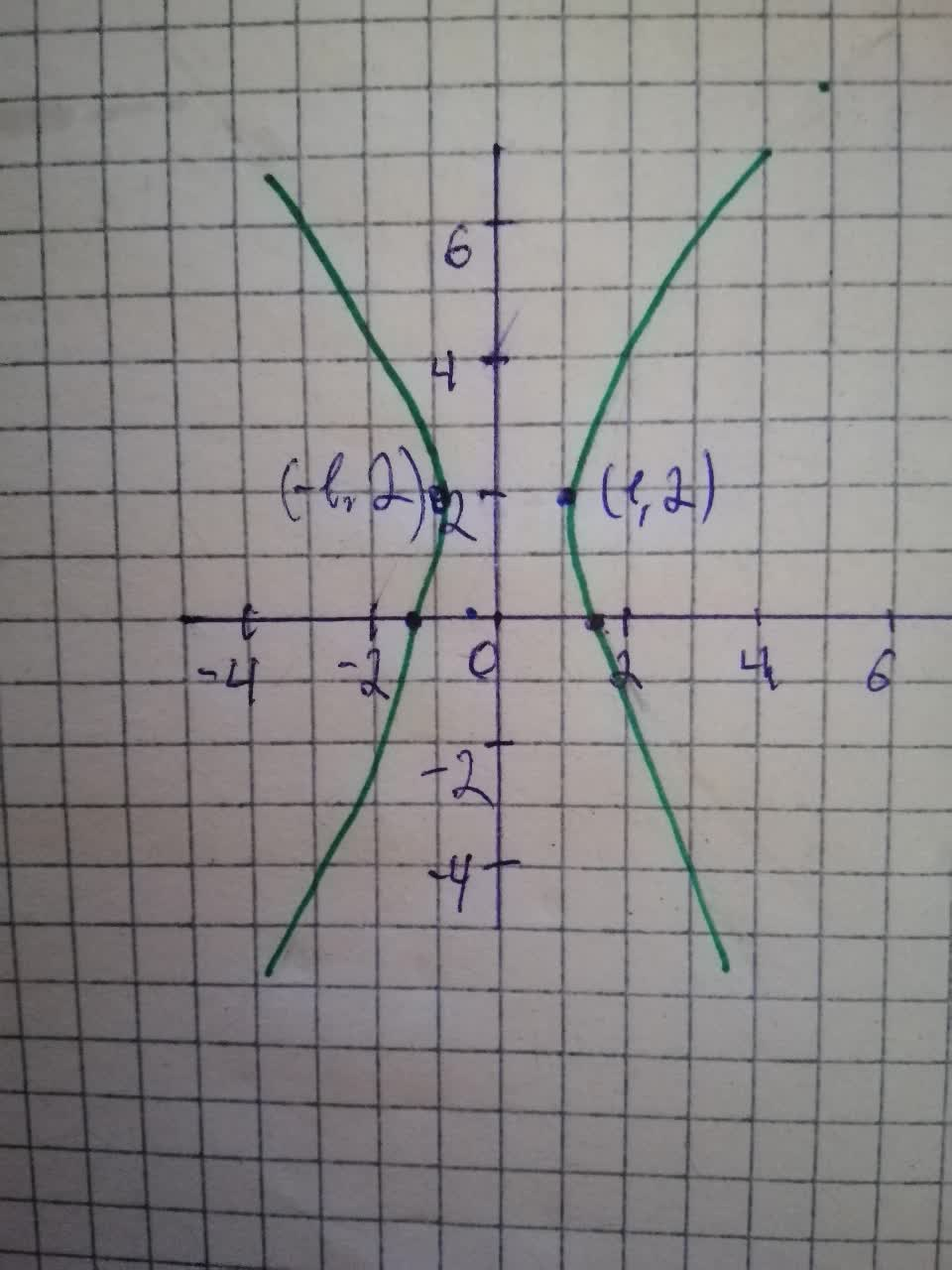# Sketch the following conic sections: 4^{2}-4y+8-4x^{2}=0smileycellist2 2021-08-15 Answered
Sketch the following conic sections:
${4}^{2}-4y+8-4{x}^{2}=0$
You can still ask an expert for help

• Questions are typically answered in as fast as 30 minutes

Solve your problem for the price of one coffee

• Math expert for every subject
• Pay only if we can solve itodgovoreh
Step 1
Given Equation: ${y}^{2}-4y+8-4{x}^{2}=0$
Converting the above equation in Standard hyperbola form standard form
$\frac{{\left(x-h\right)}^{2}}{{a}^{2}}-\frac{{\left(y-k\right)}^{2}}{{b}^{2}}=1$
The above equation in Standard form
${x}^{2}-\frac{{\left(y-2\right)}^{2}}{4}=1$
comparing with the standard form we have
Step 2
The graph of the above equation is shown below